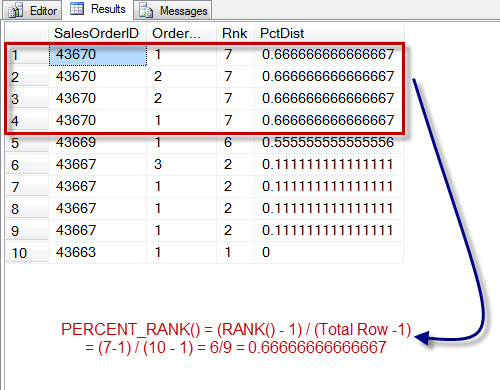# Ranking Functions

At the following picture there are 4 ranking functions at MS SQL:

1. Row_number – Numbering each row.

2. Rank – According to example below when postal code change then the function displays the value of row_number at that position.

3. Dense_Rank – According to example below when postal code change then the function increases.

4. NTile – Divide the results into groups, the example shows division to 4 groups.

You can copy and paste the following text into you MS SQL management studio if order to test it:

```SELECT     p.firstname,
p.lastname ,
Row_number() OVER (ORDER BY a.postalcode) AS “row number” ,
rank() OVER (ORDER BY a.postalcode)       AS rank ,
dense_rank() OVER (ORDER BY a.postalcode) AS “dense rank” ,
ntile(4) OVER (ORDER BY a.postalcode)     AS quartile ,
s.salesytd ,
a.postalcode
FROM       sales.salesperson AS s
INNER JOIN person.person     AS p